## Section30.6Gauss's Law Bootcamp

### Subsection30.6.3Miscellaneous

A point charge $q$ is at the center of a cube of side $a\text{.}$ Find the electric flux through one of its sides.

Hint

Think how you can use Gauss's law for closed surface.

$\dfrac{1}{6}\:\dfrac{q}{\epsilon_0}\text{.}$

Solution

By Gauss's law, flux through a closed surface equals $q_\text{enc}/\epsilon_0\text{.}$ Now, if we consider the surfaces of the cube making a closed surface around the charge at the center,we will have

\begin{equation*} \Phi_{\text{cube}} = \frac{q}{\epsilon_0}. \end{equation*}

Since the charge is at the center of the cube, we can assert that flux through each face of the cube will be same based on the symmetry.

\begin{equation*} \Phi_{\text{cube}} = 6\times \Phi_{\text{one face}}. \end{equation*}

Therefore, we will have

\begin{equation*} \Phi_{\text{one face}} = \dfrac{1}{6}\:\dfrac{q}{\epsilon_0}. \end{equation*}

A charge $q$ is placed at one of the corners of a cube of side $a\text{.}$ Find the electric flux through the shaded face shown in the figure. Assume $q>0\text{.}$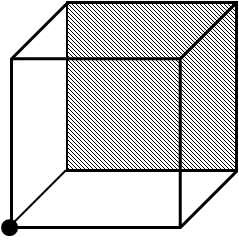Hint

Think of enclosing the charge symmetrically by a larger cube, and the, use Gauss's law on the larger cube.

$\dfrac{1}{24}\:\dfrac{q}{\epsilon_0}\text{.}$

Solution

Since the charge is at one corner of the cube, we do not have a symmetry like the one we had when the charge was located at the center of the cube in Problem 30.6.18.

However, we can imagine adding seven more cubes to form a larger cube of side $2a$ so that the charge now sits at the center of the larger cube. According to Gauss's law, the electric flux trough the surface of the larger cube will be

\begin{equation*} \Phi_{\text{larger cube}} = \dfrac{q}{\epsilon_0}. \end{equation*}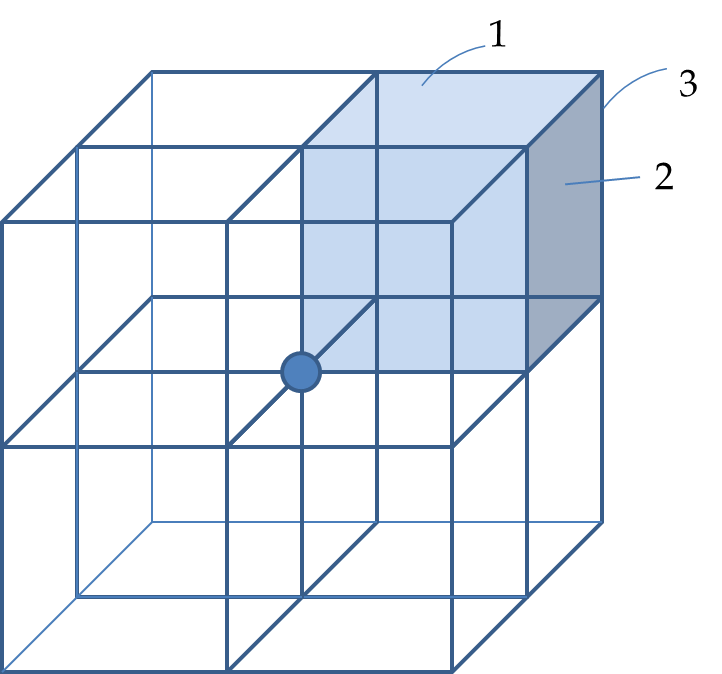Now, there are $8$ smaller cubes, each with $3$ faces in the same symmetric situation. This makes net flux equal to $8\times 3=24$ times the surface of the original face. Therefore, flux through the shaded face of the original cube will be

\begin{equation*} \Phi_{\text{larger cube}} = 24\times \Phi_{\text{shaded face original}}. \end{equation*}

Therefore, the flux through the shaded face of the original cube will be

\begin{equation*} \Phi_{\text{shaded face original}} = \dfrac{1}{24}\:\dfrac{q}{\epsilon_0}. \end{equation*}

Two non-conducting uniformly charged thin straight wires of line charge densities $\lambda_1=\lambda_0$ and $\lambda_2=-\lambda_0$ respectively are placed a distance $2d$ apart as shown in Figure 30.6.21.

Suppose the wires are parallel to the $z$ axis and cross the $xy$-plane on the $x$ axis at $x_1= -d$ and $x_2= +d\text{,}$ respectively. Find electric field at a point P in the $xy$-plane. Numerical values to use: $\lambda_0 = 10\ \mu\text{C/m}\text{,}$ $d=1.0\text{ cm}\text{,}$ $x = 2\text{ cm}\text{,}$ and $y = 4\text{ cm}\text{.}$

Hint

Use superposition of the electric field of the electric fields of the two wores. Pay attention to the directions.

$37,500\text{ N/C}$ at clockwise angle $82^\circ$ from positive $x$-axis.

Solution

I will work out the numerical problem.

Let $r_1$ and $r_2$ be the distance from the wires to the field point $(x,y)$ in the $xy$-plane .

\begin{align*} r_1 \amp = \sqrt{(x+d)^2 + y^2}, \\ \amp = \sqrt{(2+1)^2 + 4^2} = 5\text{ cm}, \\ r_2 \amp = \sqrt{(x-d)^2 + y^2}, \\ \amp = \sqrt{(2-1)^2 + 4^2} = \sqrt{17}\text{ cm}. \end{align*}

Using the formulas for the magnitudes of the electric fields from charges on long wire, which is done by Gauss's law for cyclindrical case, we get

\begin{align*} E_1 \amp = \dfrac{|\lambda_1|}{2\pi\epsilon_0}\,\dfrac{1}{r_1} \\ \amp = \dfrac{ 10\times 10^{-6}}{2\pi\times8.85\times 10^{-12}}\,\dfrac{1}{5} = 35,967\text{ N/C} \\ E_2 \amp = \dfrac{|\lambda_2|}{2\pi\epsilon_0}\,\dfrac{1}{r_2} \\ \amp = \dfrac{ 10\times 10^{-6}}{2\pi\times8.85\times 10^{-12}}\,\dfrac{1}{\sqrt{17}} = 67,972\text{ N/C} \end{align*}

Now we need to add them vectorially. The net field is not just sum of the magnitudes, as you know from vector addition.

\begin{equation*} E_P \ne E_1 + E_2. \end{equation*}

We will first compute $x$ and $y$ components of the two fields so that we can find $x$ and $y$ components of the net field.

\begin{align*} E_{1x} \amp = E_1\, \dfrac{x+d}{r_1}, \\ \amp = 35,967\text{ N/C}\, \dfrac{3}{5} = 21,580\text{ N/C}, \\ E_{1y} \amp = E_1\, \dfrac{y}{r_1}, \\ \amp = 35,967\text{ N/C}\, \dfrac{4}{5} = 28,774\text{ N/C}. \end{align*}

Similarly, we work out the components of the field of wire 2. But, here we need to multiply by $-1$ since we have a negative charge.

\begin{align*} E_{2x} \amp = E_2\, \dfrac{-(x-d)}{r_2}, \\ \amp = 67,972\text{ N/C}\, \dfrac{-1}{\sqrt{17}} = -16,486\text{ N/C}, \\ E_{2y} \amp = E_2\, \dfrac{-y}{r_2}, \\ \amp = 67,972\text{ N/C}\, \dfrac{-4}{\sqrt{17}} = -65,943\text{ N/C}. \end{align*}

Therefore, the components of the net field are

\begin{align*} E_x \amp = E_{1x} + E_{2x} = 21,580 -16,486 = 5,094\text{ N/C},\\ E_y \amp = E_{1y} + E_{2y} = 28,774 - 65,943 = -37,169\text{ N/C} \end{align*}

Therefore, the magnitude of the field is

\begin{equation*} E = \sqrt{ E_x^2 + E_y^2} = 37,500\text{ N/C}, \end{equation*}

and the direction can be given by clockwise angle from positive $x$ axis.

\begin{equation*} \theta = \tan^{-1}\left( \dfrac{-37,169}{5,094} \right) = - 82^\circ. \end{equation*}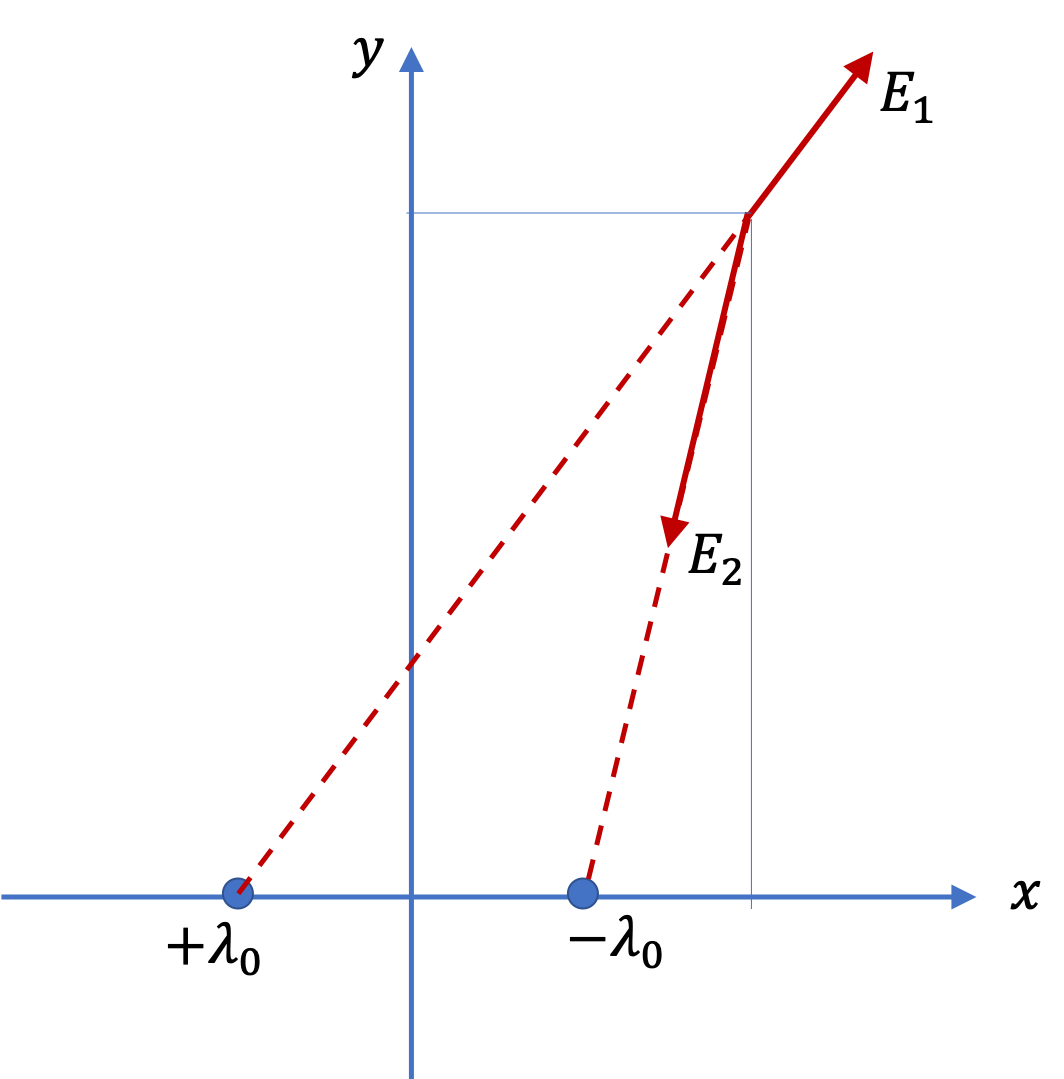A disk of radius $R$ is cut in a non-conducting large plate that is uniformly charged with charge density $\sigma$ (coulomb per square meter). Find the electric field at a height $h$ above the center of the disk. ($h>>R\text{,}$ $h\lt \lt l$ or $w$).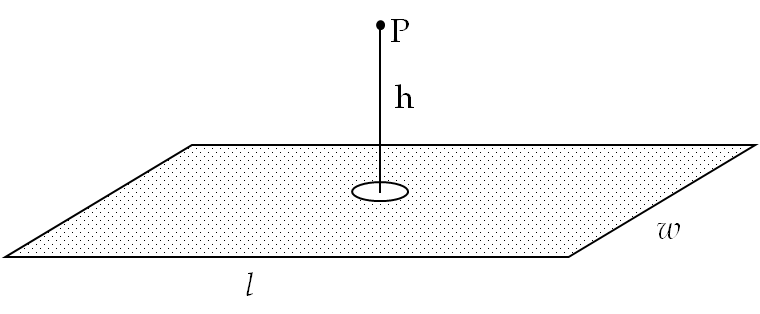Hint

Fill hole with $\pm\sigma$ and then use superposition of plane of charge and a disk.

$\dfrac{\sigma h}{2\epsilon_0\,\sqrt{R^2+h^2}}\text{.}$

Solution

Imagine filling the hole with equal and opposite charge with charge densities $+\sigma$ and $-\sigma\text{.}$ When we include the small disk of charge $+\sigma$ with the rest of the plane, we get one large plane of charge density $+\sigma\text{.}$ The charge compensating disk of charge density $-\sigma$ can be treated as a separate disk. The electric field at point P will be equal to the vector sum of the field of the entire plane and of the disk.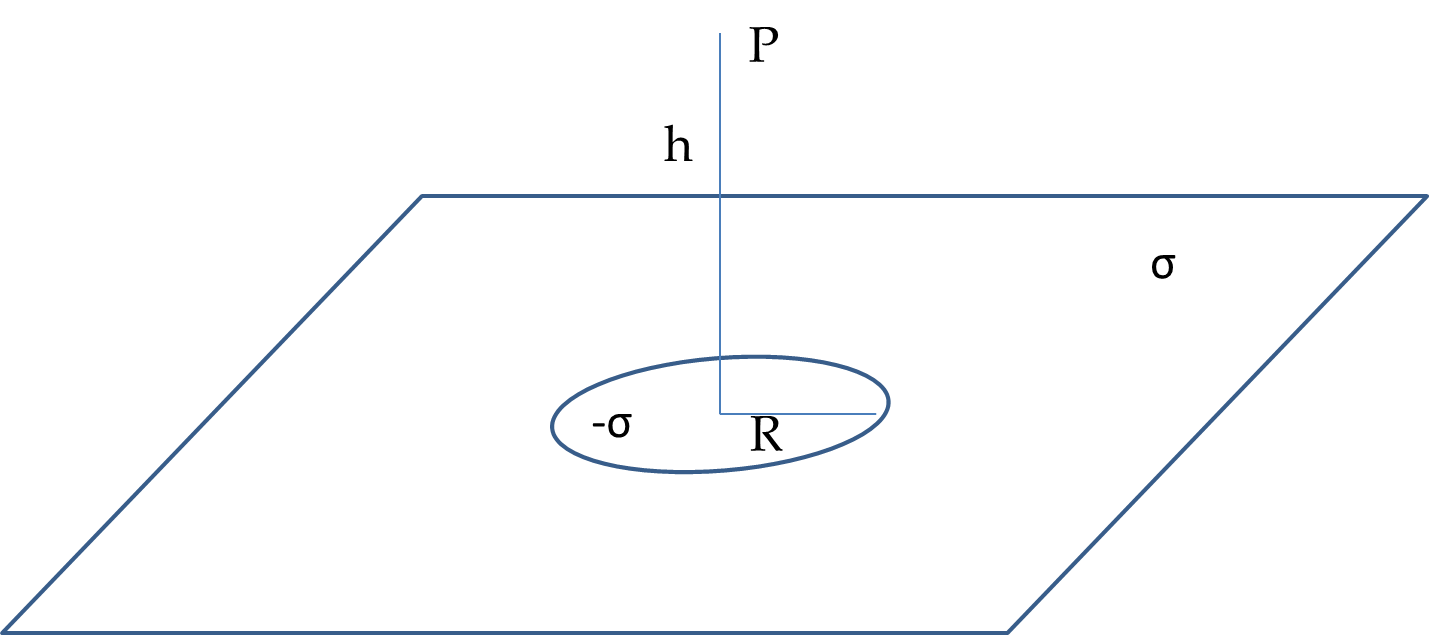We know the formulas for the electric fields of large uniformly charged plate and of a uniformly charged disk. Here the field from the plane is pointed away from the plane and the field of the disk is towards the plane.

With $z$ axis pointed up, the $z$ components of electric fields from plate and disk are

\begin{align*} E_{\text{plate},z} \amp = \dfrac{\sigma}{2\epsilon_0},\\ E_{\text{disk},z} \amp = -\dfrac{\sigma}{2\epsilon_0}\: \left( 1-\dfrac{h}{\sqrt{R^2 + h^2}} \right). \end{align*}

Adding these components of fields we obtain the $z$ component of the net electric field.

\begin{equation*} E_{z} = \dfrac{\sigma}{2\epsilon_0}\: \dfrac{h}{\sqrt{R^2 + h^2}} \end{equation*}

Since, the field has only $z$ component, this also is the magnitude of the net electric field. The direction will be away from the plate if $\sigma\gt 0$ and towards the plate if $\sigma\lt 0\text{.}$

A noncoducting sphere of radius $R$ has uniform charge density $\rho_0\text{.}$ A spherical cavity of radius $\frac{R}{2}$ is then removed, resulting in the configuration shown in Figure 30.6.24. Find electric field at points (a) $\text{P}_1\text{,}$ (b) $\text{P}_2\text{,}$ (c) $\text{P}_3\text{,}$ (d) $\text{P}_4\text{,}$ and (e) $\text{P}_5\text{.}$

Hint

Think filling the cavity with opposite charges and restoring spherical symmetry.

(a) $\frac{\rho_0 R}{6\epsilon_0}\text{,}$ left, (b) $\frac{\rho_0 R}{6\epsilon_0}\text{,}$ left, (c) $\frac{\rho_0 R}{6\epsilon_0}\text{,}$ left, (d) $\frac{17\rho_0 R}{54\epsilon_0}\text{,}$ right, (d) $\frac{5\rho_0 R}{216\epsilon_0}\text{,}$ right, (e)

Solution

As before in other problems with cavities, we will fill the cavity with opposite charges so that we can restore symmetries in charge distribution. Suppose, charge density $\rho_0$ is positive, then we will fill the cavity with positive $\rho_0$ and equal amout of negative $-\rho_0\text{.}$ Then, we include $\rho_0$ with the original charges.

This process makes two spherically symmetric charge distributions. Since we already know expressions for electric field in uniformly charged sphere, we will just use those formulas when we superimpose the answers.

We will work out directions for $\rho_0\gt 0\text{.}$ If $\rho_0\lt 0\text{,}$ you wil ljust have to flip the arrow.

(a) At $\text{P}_1\text{,}$ the field from smaller sphere will be to the right and field from the larger sphere will be to the left. This will mean they will subtract. The magnitudes of the two are such that both have contribution from all charges in their distributions since $\text{P}_1$ is outside of both - at the edge will be taken as just outside. Hence, magnitude of the field there will be

\begin{equation*} E_1 = \frac{\rho_0 R}{3\epsilon_0} - \frac{\rho_0 R/2}{3\epsilon_0} = \frac{\rho_0 R}{6\epsilon_0}. \end{equation*}

The direction is to the left.

(b) At $\text{P}_2\text{,}$ we have zero field from the smaller sphere. Since this point is an inside point for the larger sphere, we will use $E_\text{in}$ formula for that.

\begin{equation*} E_2 = \frac{\rho_0 R/2}{3\epsilon_0} = \frac{\rho_0 R}{6\epsilon_0}. \end{equation*}

The direction is to the left.

(c) At $\text{P}_3\text{,}$ we have zero field from the larger sphere. Since this point is an outside point for the smaller sphere, we will use $E_\text{out}$ formula for that. At the edge of this sphere we get

\begin{equation*} E_3 = \frac{\rho_0 R}{6\epsilon_0}. \end{equation*}

The direction is to the left.

(d) $\text{P}_4$ is outside of both spheres with larger one's field pointed to the right and smaller one's pointed left. From their superposition we get the magnitude

\begin{align*} E_4 \amp = \frac{\frac{4}{3}\pi R^3 \rho_0 }{4\pi R^2 \epsilon_0} - \frac{\frac{4}{3}\pi (R/2)^3 \rho_0 }{4\pi (3R/2)^2 \epsilon_0}\\ \amp = \frac{17}{54}\ \frac{\rho_0 R}{\epsilon_0}. \end{align*}

The direction is to the right.

(e) $\text{P}_5$ is outside of both spheres with larger one's field pointed to the right and smaller one's pointed left. From their superposition we get the magnitude

\begin{align*} E_4 \amp = \frac{\frac{4}{3}\pi R^3 \rho_0 }{4\pi (3R/2)^2 \epsilon_0} - \frac{\frac{4}{3}\pi (R/2)^3 \rho_0 }{4\pi R^2 \epsilon_0}\\ \amp = \frac{5}{216}\ \frac{\rho_0 R}{\epsilon_0}. \end{align*}

The direction is to the right.

A spherical shell of radius $R$ is charged uniformly with constant surface charge density $\sigma_0\text{.}$ A small piece of the shell is then removed, resulting in a hole in the spherical shell. Suppose the removed part is almost flat circular disk of radius $a\text{.}$ What is the electric field an infinitesimal distance $d$ above the center of the hole so that you can asume $d\lt lt a\text{?}$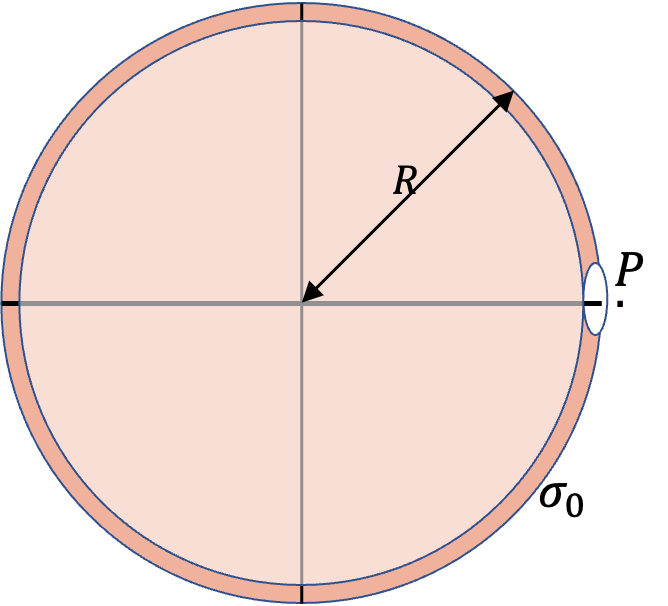You might find magnitude of electric field of a disk of radius $a$ and charge density $\sigma$ at a distance $d$ above the center to be

\begin{equation*} E = \frac{|\sigma|}{2\epsilon_0}\left( 1 - \frac{d}{\sqrt{d^2+a^2}}\right). \end{equation*}

For $d\lt\lt a\text{,}$ this simplifies to $\frac{|\sigma|}{2\epsilon_0}\text{.}$

Hint

Think filling the hole with opposite charges and restoring spherical symmetry with one and using the large sheet formula for the other.

$\sigma_0/2\epsilon_0$ radially out if $\sigma_0\gt 0\text{.}$

Solution

As before in other problems with cavities, we will fill the cavity with opposite charges so that we can restore symmetries in charge distribution. Suppose, charge density $\sigma_0$ is positive, then we will fill the cavity with positive $\sigma_0$ and equal amout of negative $-\sigma_0\text{.}$ Then, we include $\sigma_0$ with the original charges. Then, electric field at P will be a vector sum of the electric field by the spherical shell and the disk as shown in Figure 30.6.27.

Let us use a $z$ axis to take care of directions. By Gauss's law, we get electric field of the shell, which we will wrtie as $z$ component at point P.

\begin{equation*} E_{\text{shell},z} = \frac{4\pi R^2 \sigma_0}{4\pi R^2 \epsilon_0} = \frac{\sigma_0}{\epsilon_0}. \end{equation*}

Since $d \lt\lt a\text{,}$ the $z$ component of the electric field from the negative charges on the disk will be

\begin{equation*} E_{\text{disk},z} = -\frac{\sigma_0}{2\epsilon_0}. \end{equation*}

Therefore, magnitude is $\frac{\sigma_0}{2\epsilon_0}$ and direction is radially out from the center of the spherical shell.